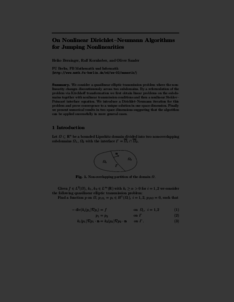Repository: Freie Universität Berlin, Math Department

On nonlinear Dirichlet-Neumann Algorithms for jumping nonlinearities. In: Domain Decomposition Methods in Science and Engineering XVI

Berninger, H. and Kornhuber, R. and Sander, O. (2007) On nonlinear Dirichlet-Neumann Algorithms for jumping nonlinearities. In: Domain Decomposition Methods in Science and Engineering XVI. In: Domain Decomposition Methods in Science and Engineering XVI. LNCSE, 55 . Springer, pp. 489-496. ISBN 978-3-540-34469-8Preview

137kB

Abstract

We consider a quasilinear elliptic transmission problem where the nonlinearity changes discontinuously across two subdomains. By a reformulation of the problem via Kirchhoff transformation we first obtain linear problems on the subdomains together with nonlinear transmission conditions and then a nonlinear Steklov–Poincar´e interface equation. We introduce a Dirichlet–Neumann iteration for this problem and prove convergence to a unique solution in one space dimension. Finally we present numerical results in two space dimensions suggesting that the algorithm can be applied successfully in more general cases.

Item Type: Book Section Mathematical and Computer Sciences > Mathematics 1710 Sabrina Nordt 24 Aug 2015 10:11 03 Mar 2017 14:41

Repository Staff Only: item control page